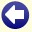## Solution to: John & Julia

The Solution: John is 30 years old and Julia is 40 years old.

An explanation:
Let Julia's age be a and let John's age be b.
The first sentence tells us, "Julia is as old as John will be when Julia is twice as old as John was when Julia's age was half the sum of their present ages".
"Half the sum of their present ages" is:
0.5*(a+b)
So "Julia's age was half the sum of their present ages", the following number of years ago:
a - (0.5*(a+b)) = 0.5*a-0.5*b
At that moment, "John's age" was:
b - (0.5*a-0.5*b) = 1.5*b-0.5*a.
So "twice that age" is:
3*b-a
"Julia's age will be twice that age", in the following number of years:
(3*b-a) - a = 3*b-2*a
At that moment, John will be:
b + (3*b-2*a) = 4*b-2*a
And we are told that "that's Julia's age", so:
a = 4*b-2*a
or
3*a = 4*b.

The second sentence tells us that "John is as old as Julia was when John was half the age he will be 10 years from now".
"10 years from now", John will be:
b+10
"Half that age" is:
0.5*b+5
"John was that age", the following number of years ago:
b - (0.5*b+5) = 0.5*b-5
At that time, "Julia was", the following number of years old:
a - (0.5*b-5) = a-0.5*b+5
And that "is John's current age", so:
b = a-0.5*b+5
or
a = 1.5*b-5.

Now we have two equations:
3*a = 4*b and a = 1.5*b-5.
Substitute a in the first equation for the value of a from the second equation and we get:
3*(1.5*b-5) = 4*b
Solving this equation, we get:
b=30
Since a = 1.5*b-5 :
a=40
Therefore, Julia's age is 40 and John's age is 30.Back to the puzzle
Copyright © 1996-2019. RJE-productions. All rights reserved. No part of this website may be published, in any form or by any means, without the prior permission of the authors.
This website uses cookies. By further use of this website, or by clicking on 'Continue', you give permission for the use of cookies. If you want more information, look at our cookie policy.